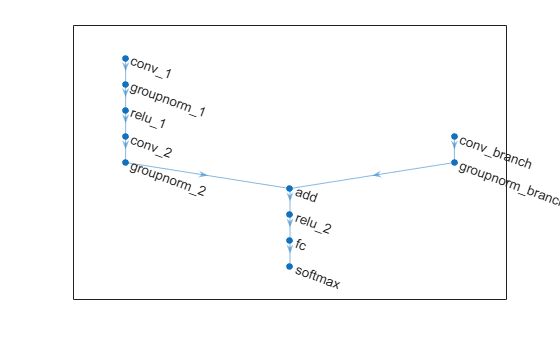# initialize

Initialize learnable and state parameters of a `dlnetwork`

## Syntax

``netUpdated = initialize(net)``
``netUpdated = initialize(net,X1,...,Xn)``

## Description

example

``` TipMost `dlnetwork` objects are initialized by default. You only need to manually initialize a `dlnetwork` if it is uninitialized. You can check if a network is initialized using the `Initialized` property of the `dlnetwork` object. `netUpdated = initialize(net)` initializes any unset learnable parameters and state values of `net` based on the input sizes defined by the network input layers. Any learnable or state parameters that already contain values remain unchanged. A network with unset, empty values for learnable and state parameters is uninitialized. You must initialize an uninitialized `dlnetwork` before you can use it. By default, `dlnetwork` objects are constructed with initial parameters and do not need initializing. ```

example

````netUpdated = initialize(net,X1,...,Xn)` initializes any unset learnable parameters and state values of `net` based on the example network inputs `X1,...,Xn`. Use this syntax when the network has inputs that are not connected to an input layer. ```

## Examples

collapse all

Define a simple image classification network as a layer array.

```layers = [ imageInputLayer([28 28 1],Normalization="none") convolution2dLayer(5,20) batchNormalizationLayer reluLayer fullyConnectedLayer(10) softmaxLayer];```

Convert the layer graph to a `dlnetwork` object. Create an uninitialized `dlnetwork` object by setting the `Initialize` option to `false`.

`net = dlnetwork(layers,Initialize=false);`

View the learnable parameters of the network. Because the network is not initialized, the values are empty.

`net.Learnables`
```ans=6×3 table Layer Parameter Value ___________ _________ ____________ "conv" "Weights" {0x0 double} "conv" "Bias" {0x0 double} "batchnorm" "Offset" {0x0 double} "batchnorm" "Scale" {0x0 double} "fc" "Weights" {0x0 double} "fc" "Bias" {0x0 double} ```

Initialize the learnable parameters of the network using the `initialize` function.

`net = initialize(net);`

View the learnable parameters of the network. Because the network is now initialized, the values are nonempty with sizes inferred using the size of the input layer.

`net.Learnables`
```ans=6×3 table Layer Parameter Value ___________ _________ ___________________ "conv" "Weights" { 5x5x1x20 dlarray} "conv" "Bias" { 1x1x20 dlarray} "batchnorm" "Offset" { 1x1x20 dlarray} "batchnorm" "Scale" { 1x1x20 dlarray} "fc" "Weights" {10x11520 dlarray} "fc" "Bias" {10x1 dlarray} ```

Define a multi-input image classification network.

```numFilters = 24; layersBranch1 = [ convolution2dLayer(3,6*numFilters,Padding="same",Stride=2) groupNormalizationLayer("all-channels") reluLayer convolution2dLayer(3,numFilters,Padding="same") groupNormalizationLayer("channel-wise") additionLayer(2,Name="add") reluLayer fullyConnectedLayer(10) softmaxLayer]; layersBranch2 = [ convolution2dLayer(1,numFilters,Name="conv_branch") groupNormalizationLayer("all-channels",Name="groupnorm_branch")]; lgraph = layerGraph(layersBranch1); lgraph = addLayers(lgraph,layersBranch2); lgraph = connectLayers(lgraph,"groupnorm_branch","add/in2"); ```

Visualize the layer graph in a plot.

```figure plot(lgraph)```Convert the layer graph to a `dlnetwork` object. Create an uninitialized `dlnetwork` object by setting the `Initialize` option to `false`.

`net = dlnetwork(lgraph,Initialize=false);`

View the learnable parameters of the network. Because the network is not initialized, the values are empty.

`net.Learnables`
```ans=14×3 table Layer Parameter Value __________________ _________ ____________ "conv_1" "Weights" {0x0 double} "conv_1" "Bias" {0x0 double} "groupnorm_1" "Offset" {0x0 double} "groupnorm_1" "Scale" {0x0 double} "conv_2" "Weights" {0x0 double} "conv_2" "Bias" {0x0 double} "groupnorm_2" "Offset" {0x0 double} "groupnorm_2" "Scale" {0x0 double} "conv_branch" "Weights" {0x0 double} "conv_branch" "Bias" {0x0 double} "groupnorm_branch" "Offset" {0x0 double} "groupnorm_branch" "Scale" {0x0 double} "fc" "Weights" {0x0 double} "fc" "Bias" {0x0 double} ```

View the names of the network inputs.

`net.InputNames`
```ans = 1x2 cell {'conv_1'} {'conv_branch'} ```

Create random `dlarray` objects representing inputs to the network. Use an example input of size 64-by-64 with 3 channels for the main branch of the network. Use an input of size 64-by-64 with 18 channels for the second branch.

```inputSize = [64 64 3]; inputSizeBranch = [32 32 18]; X1 = dlarray(rand(inputSize),"SSCB"); X2 = dlarray(rand(inputSizeBranch),"SSCB");```

Initialize the learnable parameters of the network using the `initialize` function and specify the example inputs. Specify the inputs with order corresponding to the `InputNames` property of the network.

`net = initialize(net,X1,X2);`

View the learnable parameters of the network. Because the network is now initialized, the values are nonempty with sizes inferred using the size of the input data.

`net.Learnables`
```ans=14×3 table Layer Parameter Value __________________ _________ _____________________ "conv_1" "Weights" { 3x3x3x144 dlarray} "conv_1" "Bias" { 1x1x144 dlarray} "groupnorm_1" "Offset" { 1x1x144 dlarray} "groupnorm_1" "Scale" { 1x1x144 dlarray} "conv_2" "Weights" { 3x3x144x24 dlarray} "conv_2" "Bias" { 1x1x24 dlarray} "groupnorm_2" "Offset" { 1x1x24 dlarray} "groupnorm_2" "Scale" { 1x1x24 dlarray} "conv_branch" "Weights" { 1x1x18x24 dlarray} "conv_branch" "Bias" { 1x1x24 dlarray} "groupnorm_branch" "Offset" { 1x1x24 dlarray} "groupnorm_branch" "Scale" { 1x1x24 dlarray} "fc" "Weights" {10x24576 dlarray} "fc" "Bias" {10x1 dlarray} ```

## Input Arguments

collapse all

Uninitialized network, specified as a `dlnetwork` object.

Example network inputs, specified as `dlarray` objects.

Example inputs must be formatted `dlarray` objects. Provide example inputs in the same order as the order specified by the `InputNames` property of the input network.

## Output Arguments

collapse all

Initialized network, returned as a `dlnetwork` object.

## Version History

Introduced in R2021a